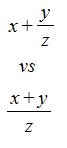# Symbolic Expressions - Overview

Expressions are created using objects, for example, Equal, Add and Sub. The objects you can use to build expressions are defined in the libraries loaded into the current document.

A symbolic expression (or just "expression") is built from instances of one or more objects. So the expression

x = 1

contains the Equal object, the "x" object and the "1" object and would be written as "Equal[x, 1]" without any rendering applied. See the Expression Rendering section for more information about rendering of expressions.

Similarly, the expression

x = y + 1

would be written as Equal[x, Add[y, 1]] without any rendering.

Symbolic expressions are just a hierarchy, or tree, of symbolic objects. When we create, highlight and manipulate expressions, we are doing things that act on one (or more) of the objects somewhere in the expression tree.

The number of parameters an object can have and what objects can populate those parameters is determined by the type definition of the object. See the Types - Overview section for more details.

With some basic understanding of what expressions are, we can now construct some simple expressions. When constructing expressions, the highlighting plays an important role. For example, the following expressions are mathematically different and which one you end up with depends on the highlighting when you create the subtraction object.To create 2(x-y):

1. Double-click to create an empty box.
2. Type 2*x to create the expression 2x. At this point, only the x in the expression is highlighted.
3. Type the minus sign followed by y to create 2(x-y). The parentheses are automatically added.

To create 2x-y:

1. Double-click to create an empty box.
2. Type 2*x to create the expression 2x. At this point, only the x in the expression is highlighted.
3. Press the up arrow to highlight 2x, then type the minus sign followed by y.

Note that without any rendering, the first expression would be Mult[2, Sub[x, y]] while the second expression would be Sub[Mult[2, x], y]. When we use commands that create objects (the "minus" character in this example) the highlighted term normally ends up as the first parameter of the new object.

Similarly, which of these two expressions is created depends on whether you just have "y" highlighted or whether you have "x + y" highlighted when you create the division object using the "/" keystroke:We will often refer to objects just by name - for example, the Equal object. But you should be aware that the full name of an object is determined by both a name and a namespace. The Equal object was created in the Math namespace and we would write the name as Math.Equal if we needed to distinguish it from an Equal object defined in another namespace. One of the main reasons for using namespaces is to prevent name collisions between objects defined in different libraries.